# 3-Card Poker Hands

Brian Alspach

13 January 2000

### Abstract:

We determine the number of 3-card poker hands.

The types of 3-card poker hands are

• straight flush
• 3-of-a-kind
• straight
• flush
• a pair
• high card

The total number of 3-card poker hands is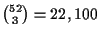.

A straight flush is completely determined once the smallest card in the straight flush is known. There are 48 cards eligible to be the smallest card in a straight flush. Hence, there are 48 straight flushes.

In forming a 3-of-a-kind hand, there are 13 choices for the rank and 4 choices for the 3 cards of the given rank. This implies there are3-of-a-kind hands.

The ranks of the cards in a straight have the form x,x+1,x+2, where x can be any of 12 ranks. There are then 4 choices for each card of the given ranks. This yields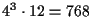total choices. However, this count includes the straight flushes. Removing the 48 straight flushes leaves us with 720 straights.

To count the number of flushes, we obtain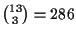choices for 3 cards in the same suit. Of these, 12 are straight flushes whose removal leaves 274 flushes of a given suit. Multiplying by 4 produces 1,096 flushes.

Now we count the number of hands with a pair. There are 13 choices for the rank of the pair, and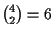pairs of the chosen rank. The non-pair card can be any of the remaining 48. Thus, there are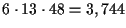3-card hands with a single pair.

We could determine the number of high card hands by removing the hands which have already been counted in one of the previous categories. Instead, let us count them independently and see if the numbers sum to 22,100 which will serve as a check on our arithmetic.

A high card hand has 3 distinct ranks, but does not allow ranks of the form x,x+1,x+2 as that would constitute a straight. Thus, there arepossible sets of ranks from which we remove the 12 sets of the form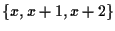. This leaves 274 sets of ranks. For a given set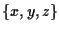of ranks, there are 4 choices for each card except we cannot choose all in the same suit. Hence, there are 274(43-4) = 16,440 high card hands.

If we sum the preceding numbers, we obtain 22,100 and we can be confident the numbers are correct.

Here is a table summarizing the number of 3-card poker hands. The probability is the probability of having the hand dealt to you when dealt 3 cards.

 hand number Probability straight flush 48 .0022 3-of-a-kind 52 .0024 straight 720 .0326 flush 1,096 .0496 pair 3,744 .1694 high card 16,440 .7439

Home | Publications | Preprints | MITACS | Poker Digest | Graph Theory Workshop | Poker Computations | Feedback
website by the Centre for Systems Science
last updated 13 January 2000